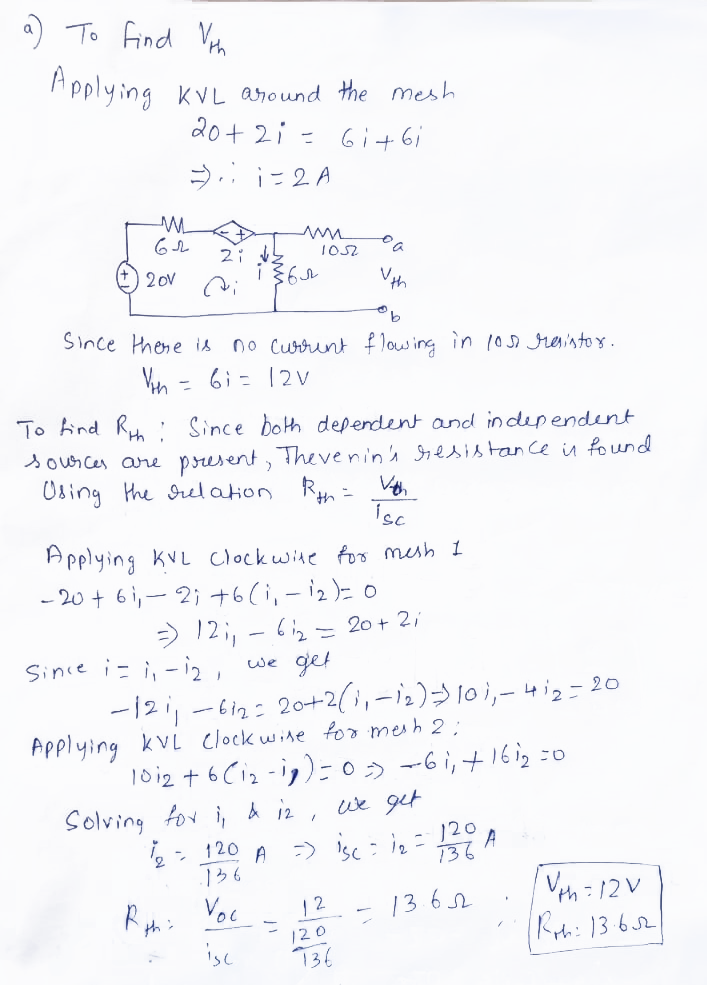### Thevenin’s Theorem

Thevenin’s Theorem

According to Thevenin's theorem, any linear circuit, no matter how complex, can be simplified to an equivalent circuit with a single voltage source (Vth)  and a series resistance (Rth).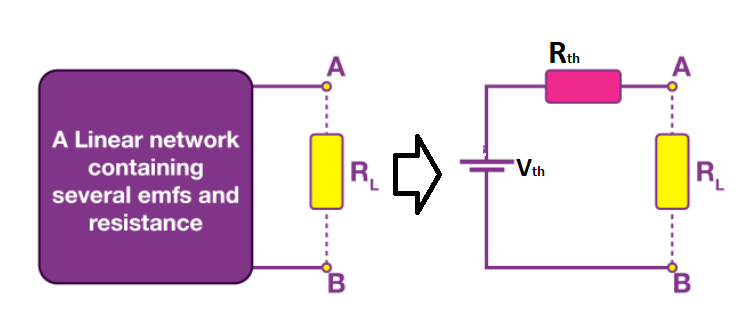Procedure to find Vth and Rth

• Remove the load resistor from the circuit.

• Measure open circuit voltage(Thevenin Voltage Vth).

• Short voltage sources and open current sources.

• Measure the Open Circuit Resistance (Thevenin Resistance Rth).

• Redraw the circuit with the measured open circuit voltage (Vth) in Step (2) as the voltage source and the measured open circuit resistance (Rth) in Step (4) as the series resistance, and reconnect the load resistor we removed in Step 1. This is the Thevenin circuit equivalent of the linear electric network or complex circuit.

• Using Ohm's Law, calculate the total current flowing through the load resistor: Iload=VthR+Rth

Example 1: Obtain Thevenin’s Equivalent of network shown below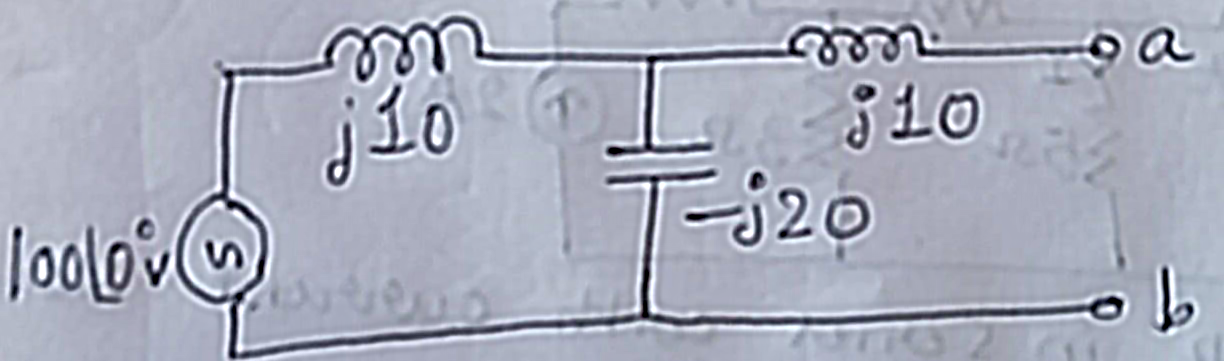Solution: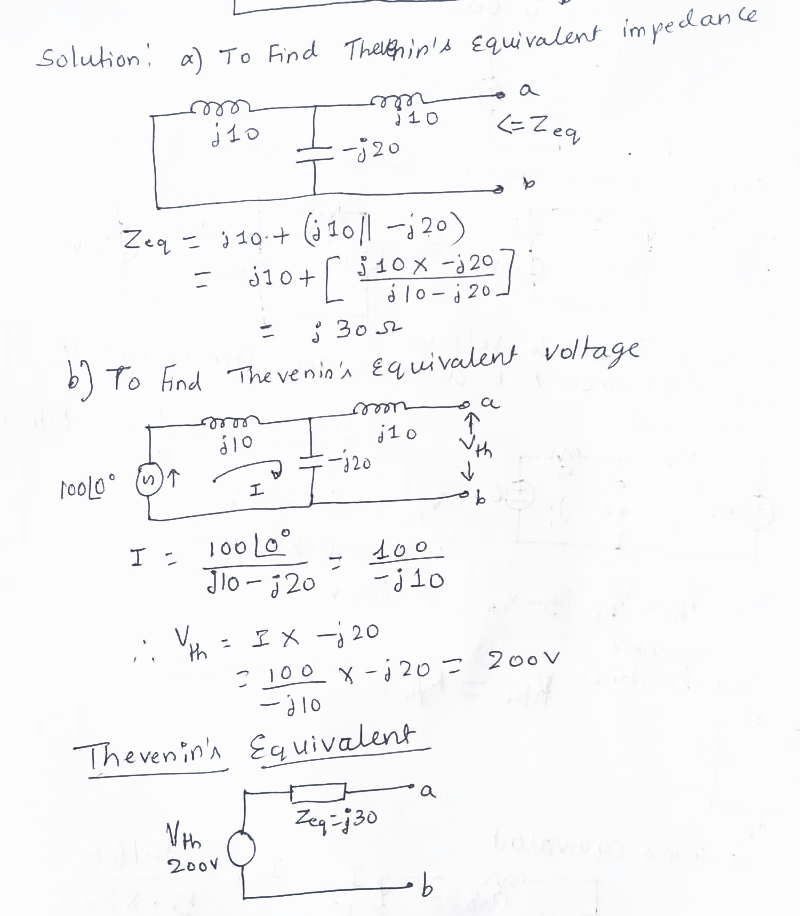Example 2: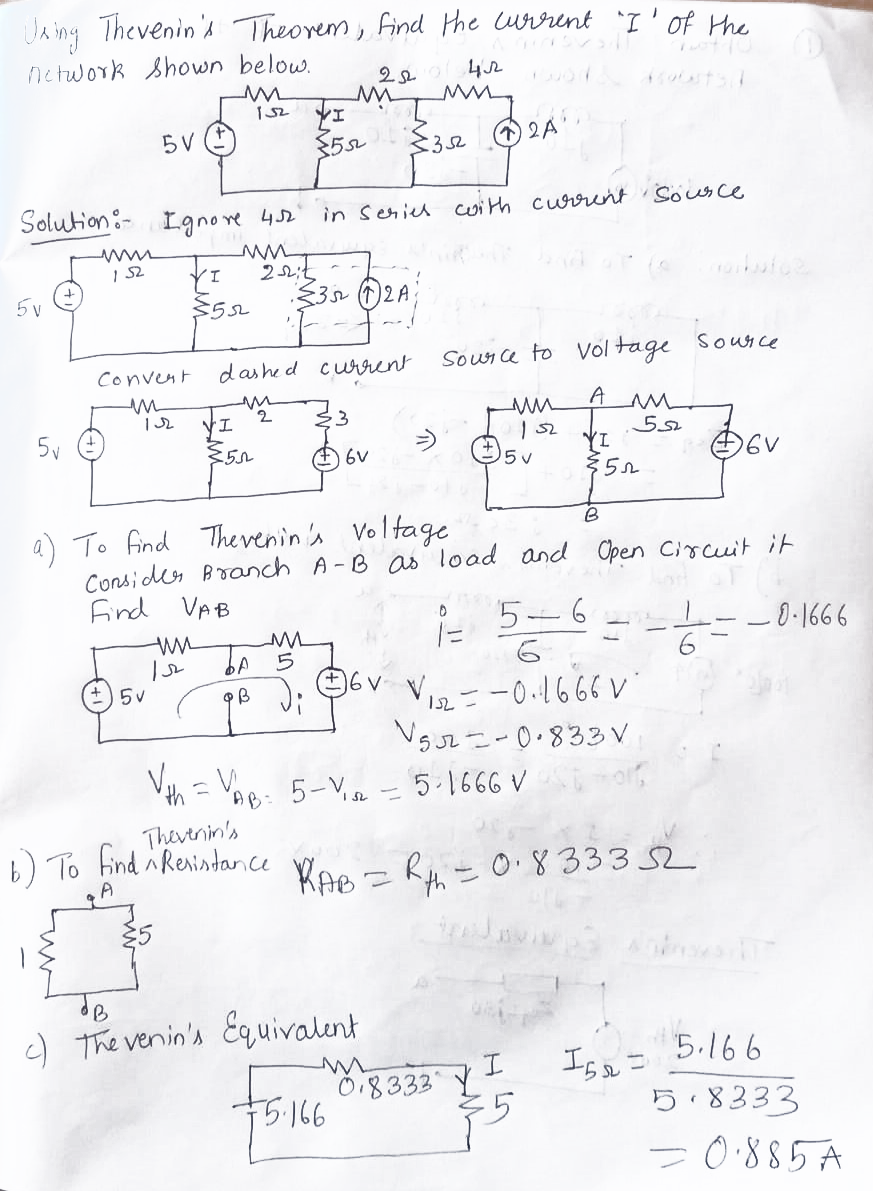Example 3: A Wheatstone bridge network is shown in Figure 13.38. Calculate the current flowing in the 10 resistor using Thévenin’s theorem.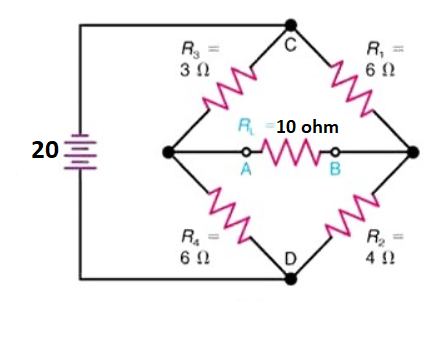Solution: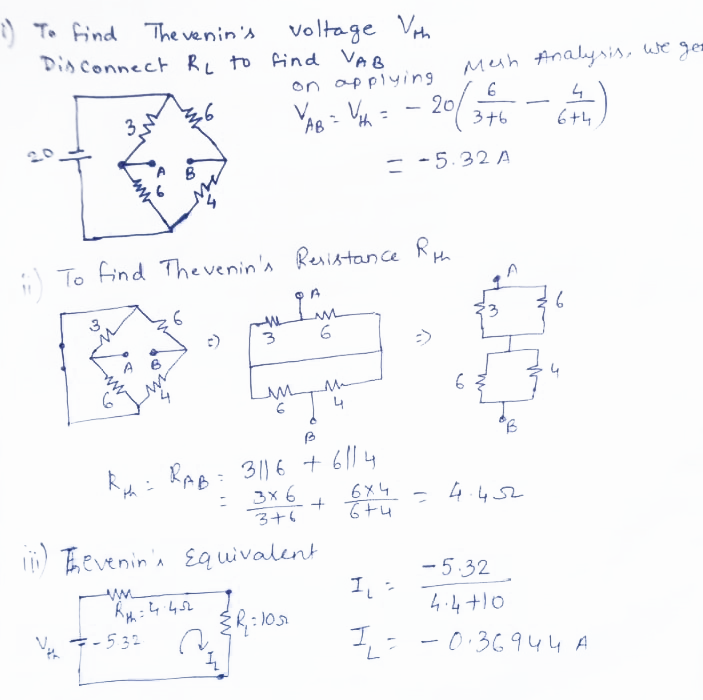Example 4: Determine V0 using Thevenin’s Theorem.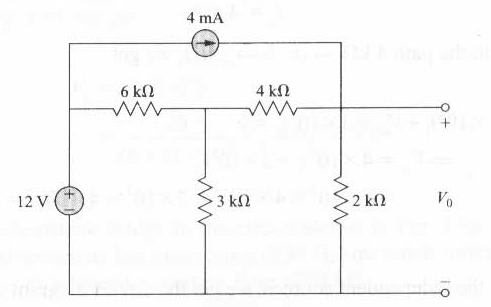Solution: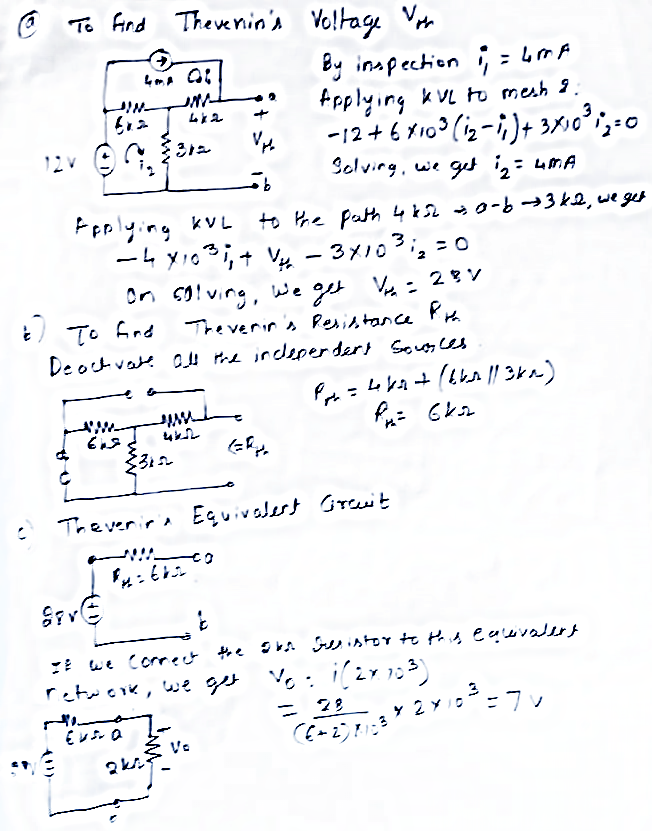Example 5: Obtain Thevenin’s Equivalent for the circuit shown below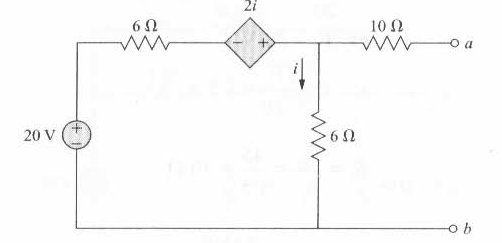Solution: